# Dice Reasoning Practice Questions: Level 01

Q.1. When the given figure is folded to form a cube then which face is opposite to the face with 2?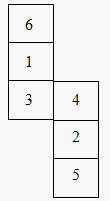A. 2
B. 4
C. 1
D. 6
Q.2. Choose a box which is similar to the box formed from the given sheet of paper.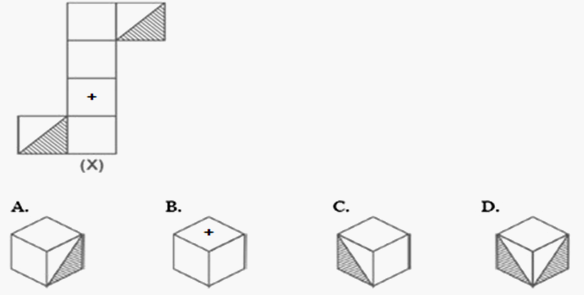A. B & D only
B. A & D only
C. A & C only
D. C & D only
Q.3. Choose a box that is similar to the box formed from the given sheet of paper.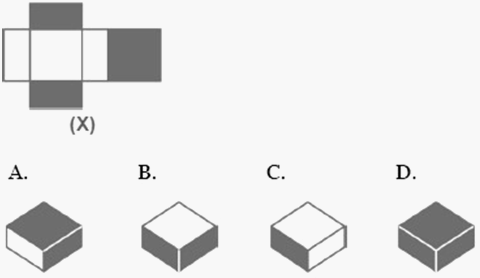A. A & C only
B. A & D only
C. B & C only
D. C & D only
Directions for Question 4 to 10: A sheet of paper shown in the figure (X) given on the leftmost side, in each given question, is folded to form a box. Choose from amongst the alternatives (1), (2), (3) and (4), the boxes that are similar to the box that will be formed.
Q.4. Choose a box that is similar to the box formed from the given sheet of paper (X).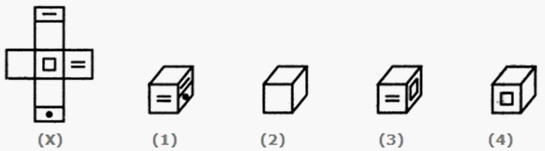A. 1 and 2 only
B. 1,2 and 3 only
C. 1, 3 and 4 only
D. 1, 2, 3 and 4
Q.5. Choose a box that is not different to the box formed from the given sheet of paper (X).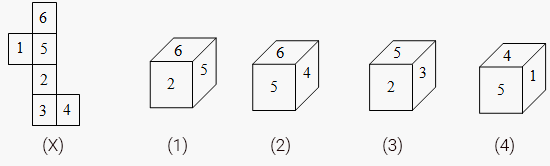A. 1 and 2 only
B. 2 only
C. 1, 2 and 3 only
D. 1, 2, 3 and 4 only
Q.6. Choose a box that is similar to the box formed from the given sheet of paper (X).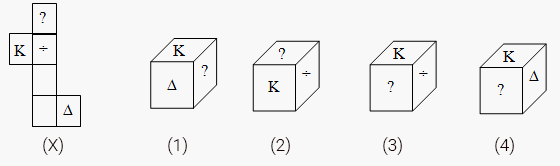A. 2 only
B. 3 only
C. 1 only
D. 4 only
Q.7. Choose a box that is similar to the box formed from the given sheet of paper (X).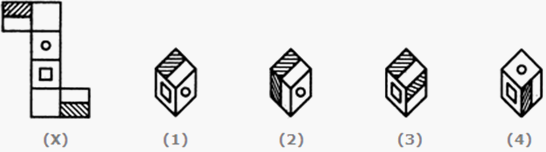A. 3 only
B. 2 only
C. 1 only
D. 4 only
Q8. Choose a box that is similar to the box formed from the given sheet of paper.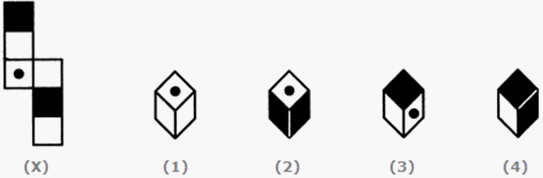A. 1,2 and 3 only
B. 1, 3 and 4 only
C. 1,2 and 4 only
D. 2 ,3 and 4 only
Q9. Choose a box that is similar to the box formed from the given sheet of paper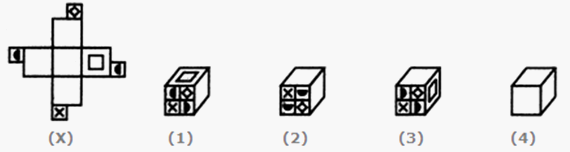A. 1, 3 and 4 only
B. 1, 2 and 3 only
C. 1, 2 and 4 only
D. 2, 3 and 4 only
Q10. Choose a box that is similar to the box formed from the given sheet of paper.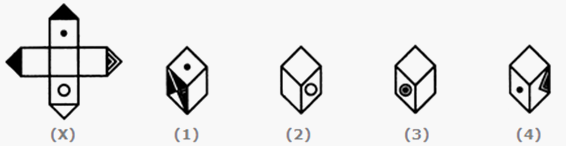A. 1 and 3 only
B. 1 ,2 and 4 only
C. 1 and 2 only
D. 1, 2, 3 and 4